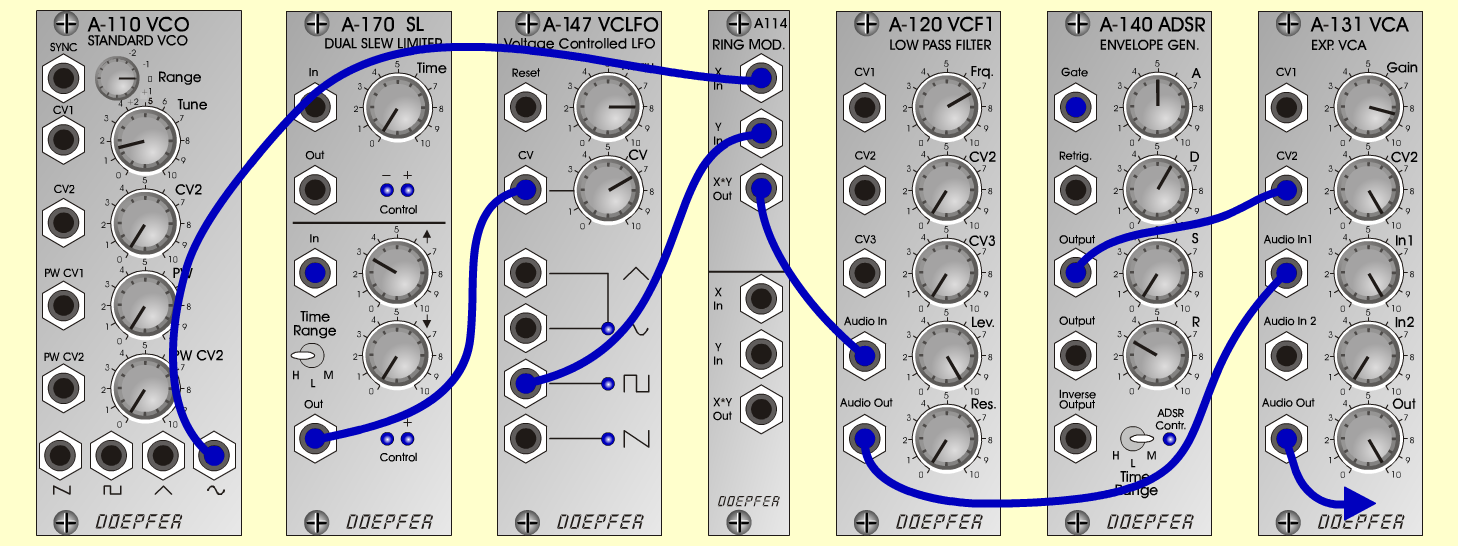RacecarFXD o e p f e r A - 100 Connections: Settings: A-110 (Sine)  <=>  A-114 (X In) KB trigger and gate  <=>  A-180 A-180   <=>  A-170/2 (In) A-170/2 (Out)  <=>  A-147 (CV) A-147 (Square)  <=>  A-114 (Y In) A-114 (Out)  <=>  A-120 (Audio In) A-120 (Audio Out)  <=>  A-131 (Audio In) A-180  <=>  A-131 (CV 1) A-131 (Audio Out)  <=> Amplifier A-110 (Range = 0, Tune = 1.5) A-170 (/\ = 3, \/ = 0, Range = H) A-120 (Freq = 7, Lev = 10, Res = 0) A-147 (Freq = 8, CV = 7) A-140 (A = 5, D = 6, S = 0, R = 3, Range = M) A-131 (Gain = 8.5, CV 2 = 10, Audio In 1 = 10,  Audio Out = 10) Notes: A-147:  If you don't have the modul, take the A-145 and turn up and down the fc-Pot manually. KB-Trigger/Gate    no: idle motion,    short: step on the gas,    hold: drive. Josef MuellerSound sample Racecar